# Selina Solutions Concise Maths Class 7 Chapter 22: Probability

Selina Solutions Concise Maths Class 7 Chapter 22 Probability has answers curated by subject matter experts at BYJU’S. The solutions are explained in a comprehensive manner to provide the students with a better conceptual knowledge. Students can make use of solutions PDF to get their doubts cleared instantly. Here, the students can download, Selina Solutions Concise Maths Class 7 Chapter 22 Probability PDF, from the links which are given below.

Chapter 22 explains the concept of experimental approach, empirical probability and complementary events. PDF can be used by the students to verify their answers and analyse their hold on the concepts.

## Selina Solutions Concise Maths Class 7 Chapter 22: Probability Download PDF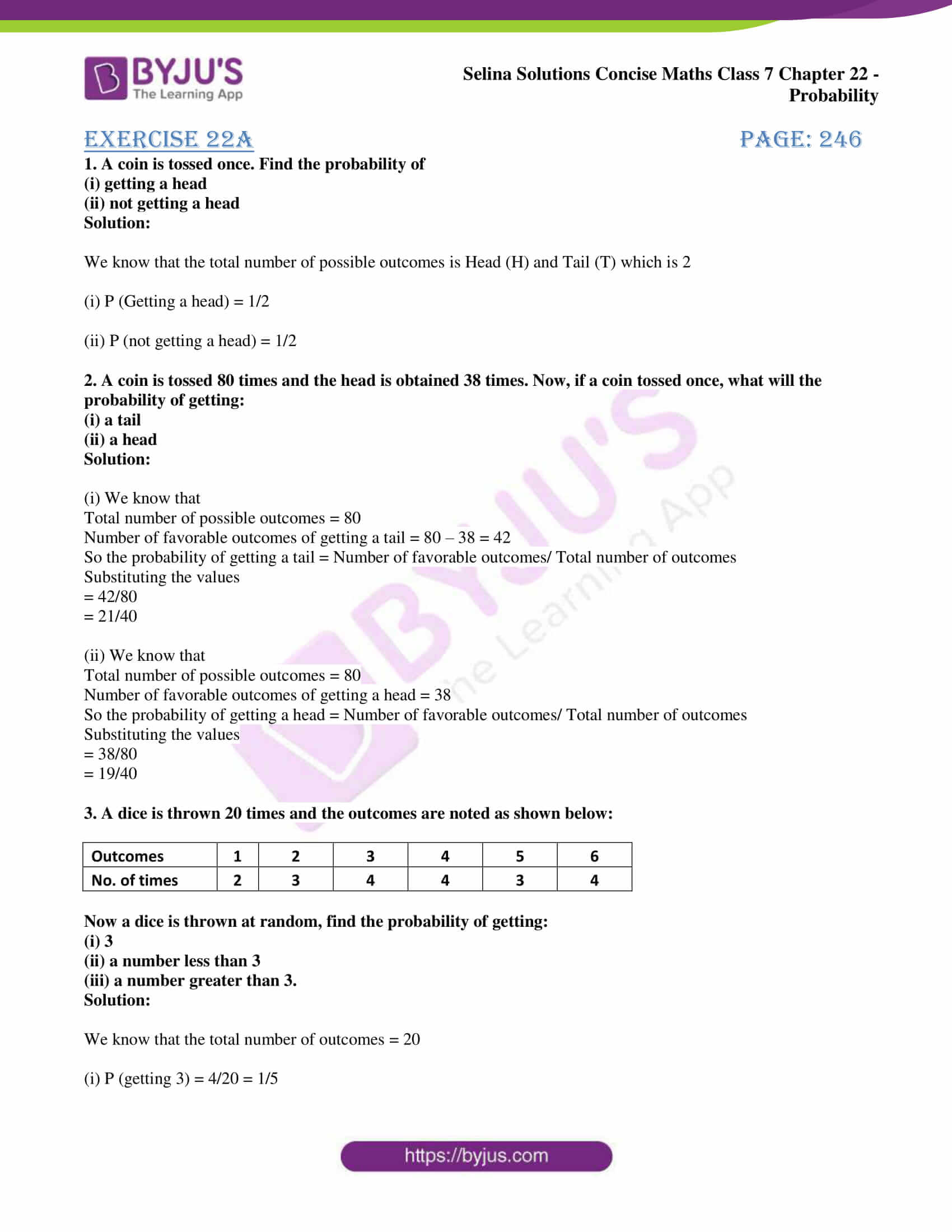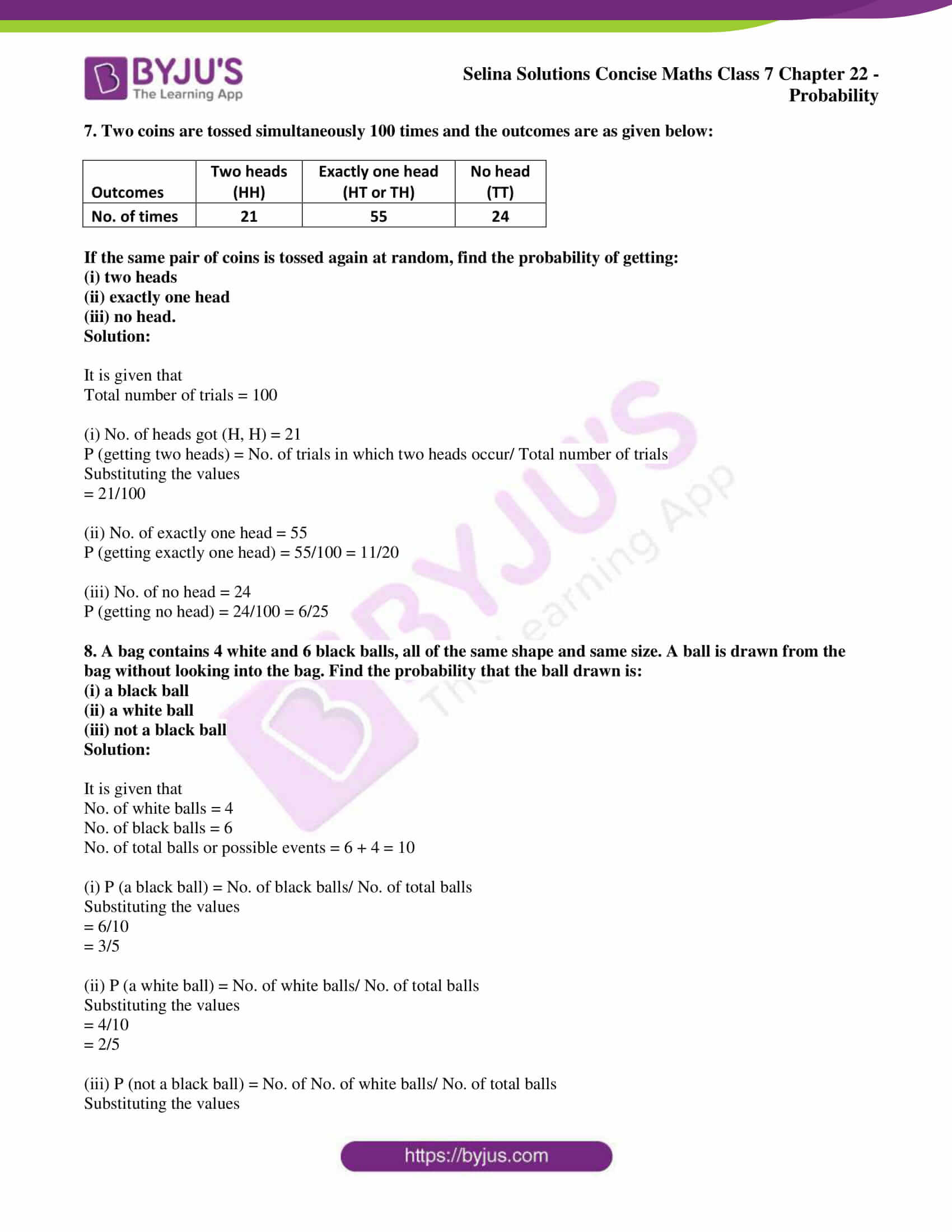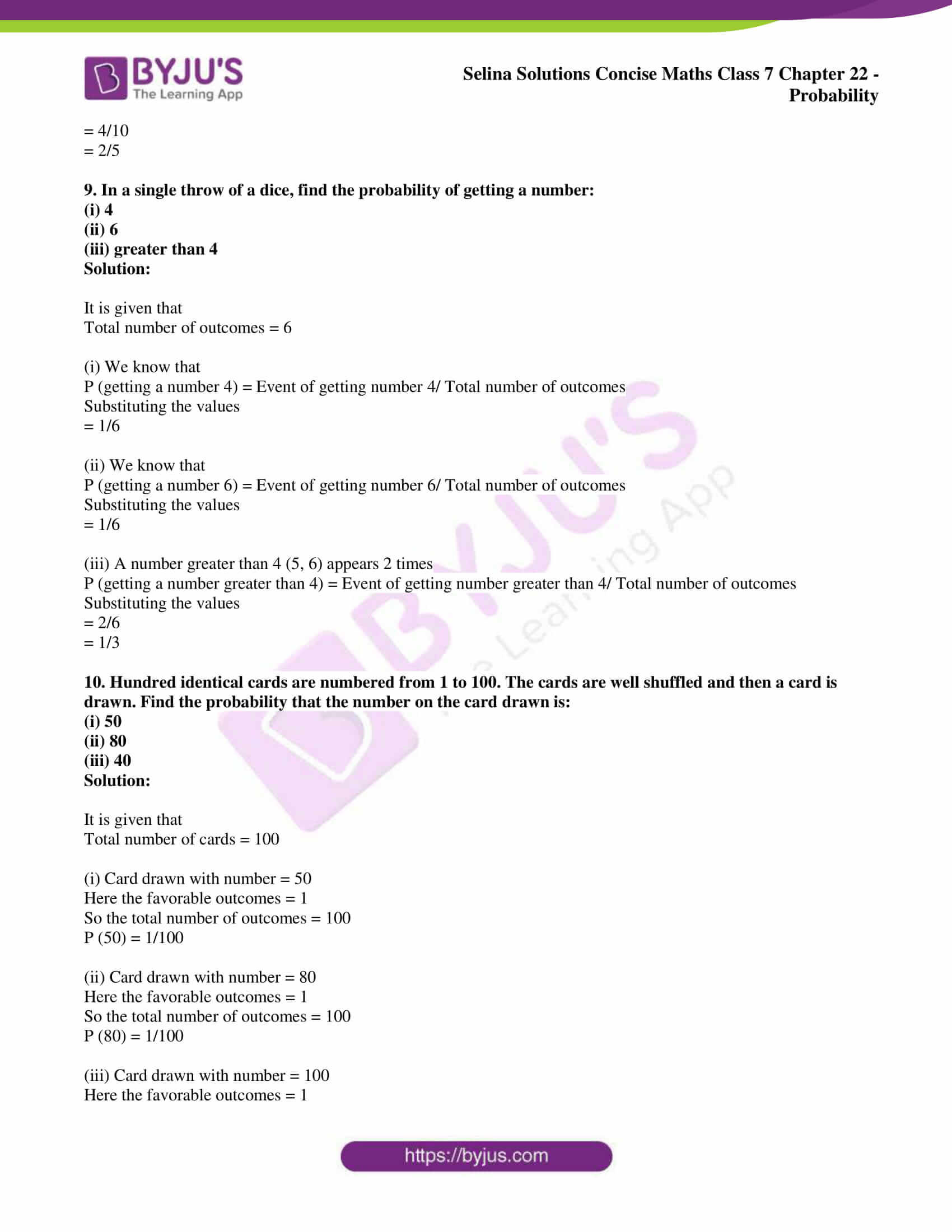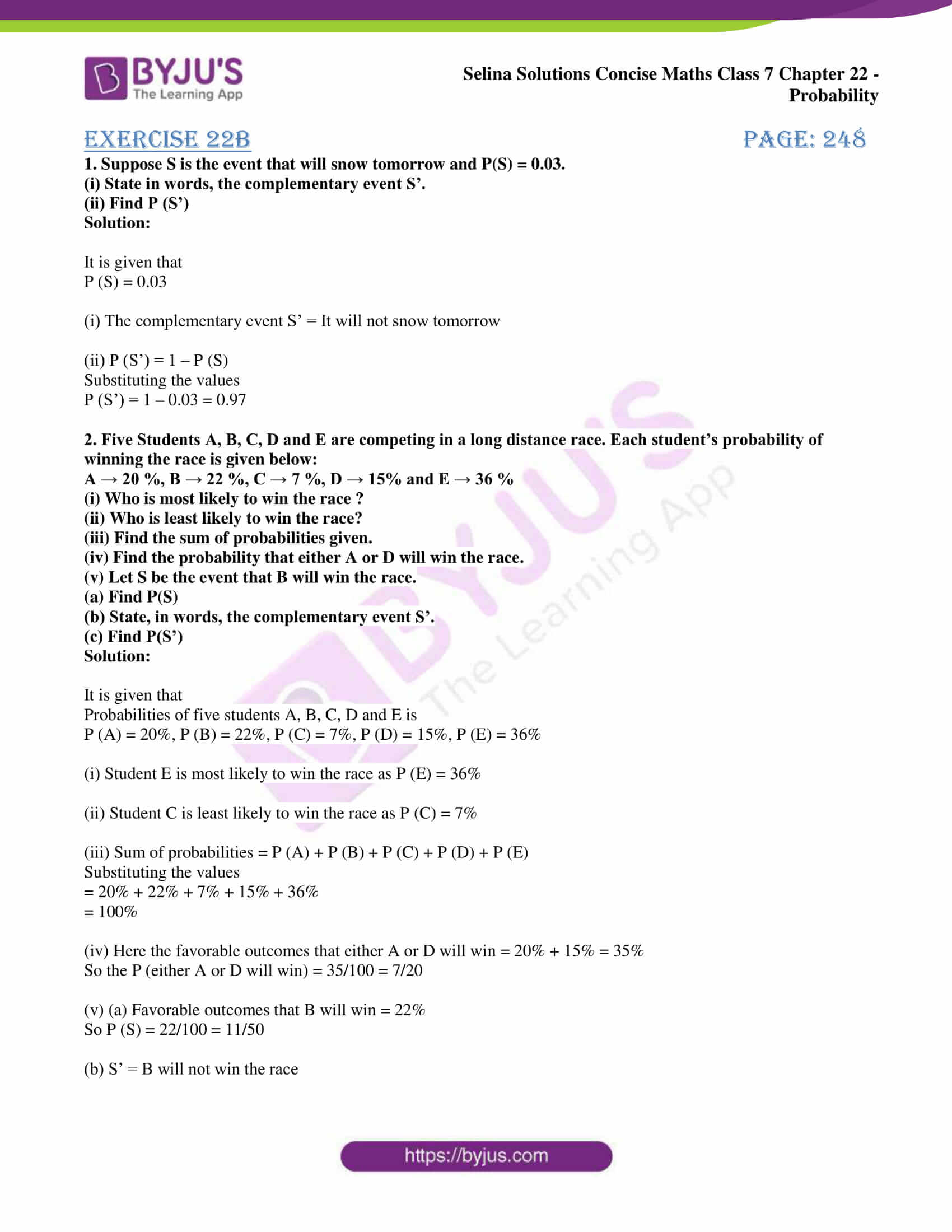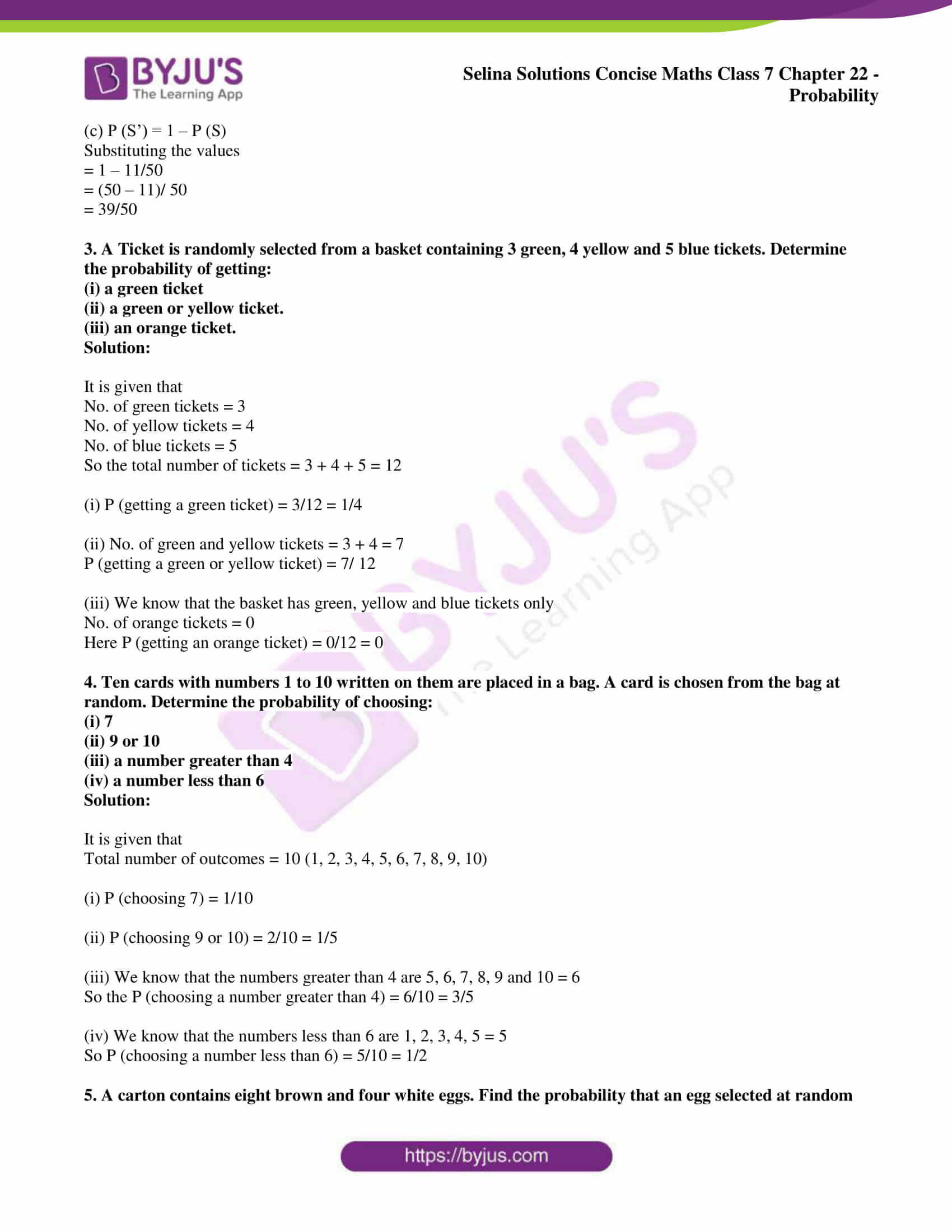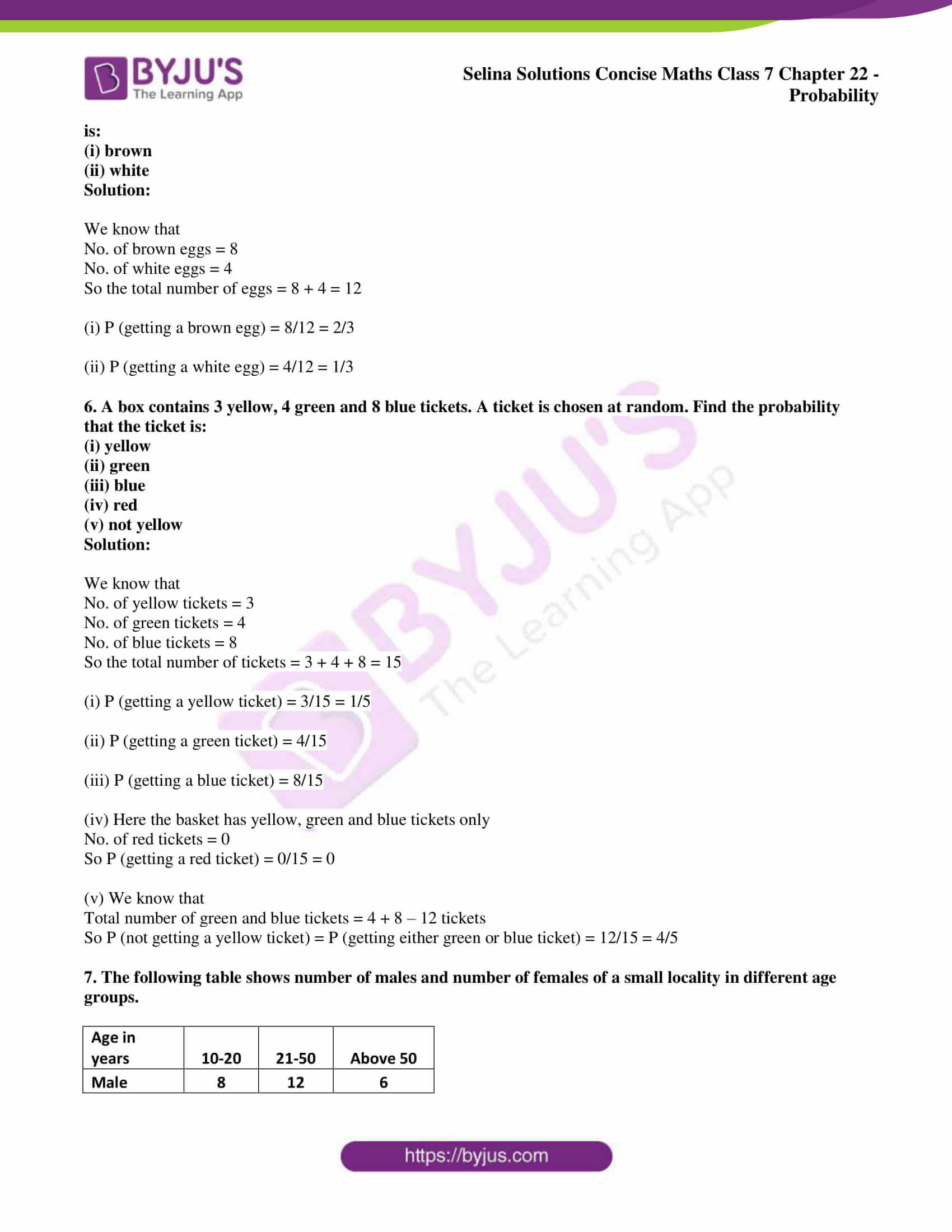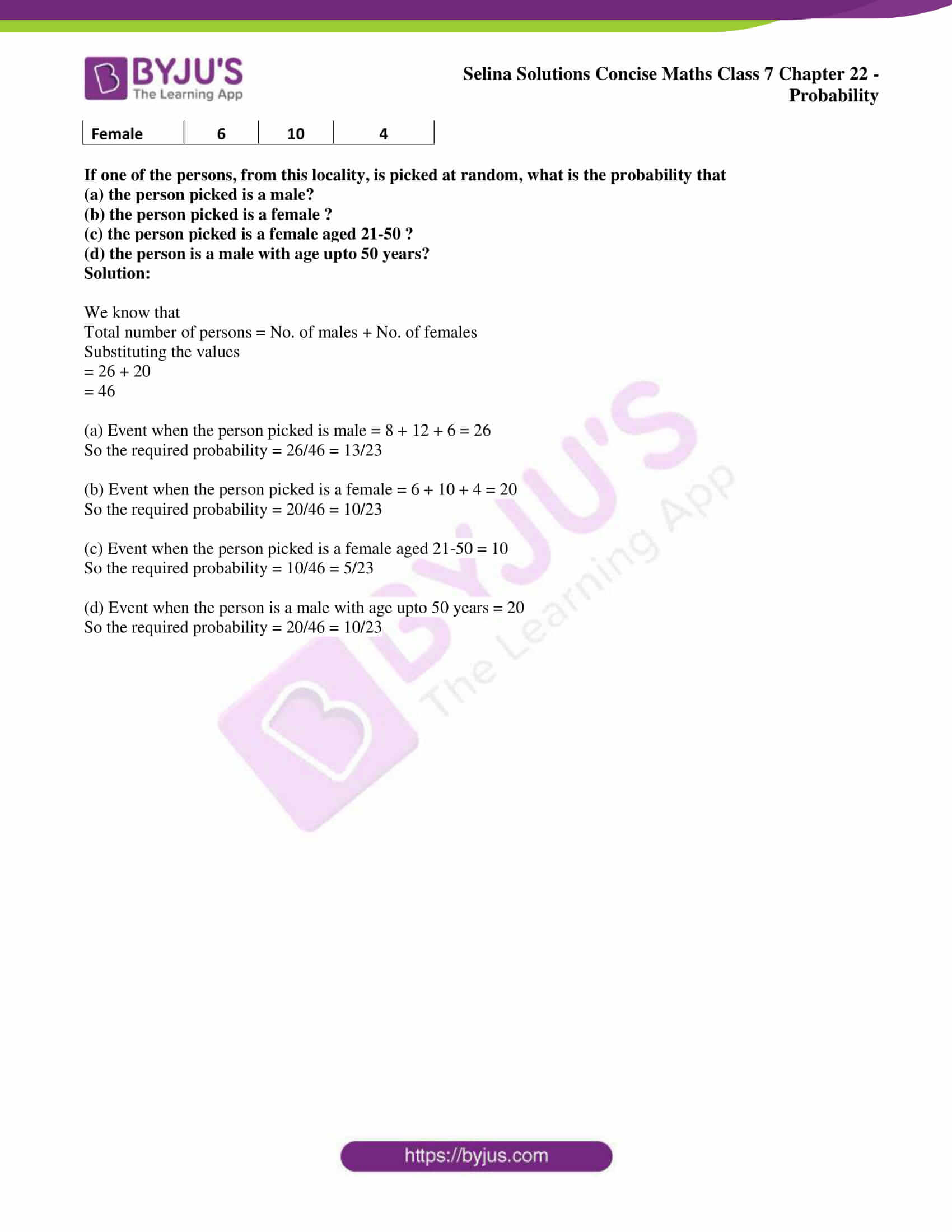## Access Selina Solutions Concise Maths Class 7 Chapter 22: Probability

#### Exercise 22A page: 246

1. A coin is tossed once. Find the probability of

Solution:

We know that the total number of possible outcomes is Head (H) and Tail (T) which is 2

(i) P (Getting a head) = 1/2

(ii) P (not getting a head) = 1/2

2. A coin is tossed 80 times and the head is obtained 38 times. Now, if a coin tossed once, what will the probability of getting:

(i) a tail

Solution:

(i) We know that

Total number of possible outcomes = 80

Number of favorable outcomes of getting a tail = 80 – 38 = 42

So the probability of getting a tail = Number of favorable outcomes/ Total number of outcomes

Substituting the values

= 42/80

= 21/40

(ii) We know that

Total number of possible outcomes = 80

Number of favorable outcomes of getting a head = 38

So the probability of getting a head = Number of favorable outcomes/ Total number of outcomes

Substituting the values

= 38/80

= 19/40

3. A dice is thrown 20 times and the outcomes are noted as shown below:

 Outcomes 1 2 3 4 5 6 No. of times 2 3 4 4 3 4

Now a dice is thrown at random, find the probability of getting:

(i) 3

(ii) a number less than 3

(iii) a number greater than 3.

Solution:

We know that the total number of outcomes = 20

(i) P (getting 3) = 4/20 = 1/5

(ii) A number less than 3

(1, 2) will appear = 2 + 3 = 5 times

So probability = 5/20 = ¼

(iii) A number greater than 3

(4, 5, 6) will appear = 4 + 3 + 4 = 11 times

So probability = 11/20

4. A survey of 50 boys showed that 21 like tea while 29 dislike it. Out of these boys, one boy is chosen at random. What is the probability that the chosen boy
(i) likes tea
(ii) dislikes tea

Solution:

It is given that

Total number of boys = 50

No. of boys who like tea = 21

No. of boys who dislike tea = 29

(i) Probability of boys who like tea = 21/50

(ii) Probability of boys who dislike tea = 29/50

5. In a cricket match, a batsman hits a boundary 12 times out of 80 balls he plays. Further, if he plays one ball more, what will be the probability that:

(i) he hits a boundary

(ii) he does not hit a boundary

Solution:

It is given that

Total number of balls = 80

No. of times batsman hits a boundary = 12

(i) P (he hits a boundary) = 12/80 = 3/20

(ii) P (he does not hit a boundary) = 68/80 = 17/20

6. There are 8 marbles in a bag with numbers from 1 to 8 marked on each of them. What is the probability of drawing a marble with number

(i) 3

(ii) 7

Solution:

It is given that

Total number of marbles = 8

(i) P (drawing a marble with number 3) = 1/8

(ii) P (drawing a marble with number 7) = 1/8

7. Two coins are tossed simultaneously 100 times and the outcomes are as given below:

 Outcomes Two heads (HH) Exactly one head (HT or TH) No head (TT) No. of times 21 55 24

If the same pair of coins is tossed again at random, find the probability of getting:

Solution:

It is given that

Total number of trials = 100

(i) No. of heads got (H, H) = 21

P (getting two heads) = No. of trials in which two heads occur/ Total number of trials

Substituting the values

= 21/100

(ii) No. of exactly one head = 55

P (getting exactly one head) = 55/100 = 11/20

(iii) No. of no head = 24

P (getting no head) = 24/100 = 6/25

8. A bag contains 4 white and 6 black balls, all of the same shape and same size. A ball is drawn from the bag without looking into the bag. Find the probability that the ball drawn is:

(i) a black ball

(ii) a white ball

(iii) not a black ball

Solution:

It is given that

No. of white balls = 4

No. of black balls = 6

No. of total balls or possible events = 6 + 4 = 10

(i) P (a black ball) = No. of black balls/ No. of total balls

Substituting the values

= 6/10

= 3/5

(ii) P (a white ball) = No. of white balls/ No. of total balls

Substituting the values

= 4/10

= 2/5

(iii) P (not a black ball) = No. of No. of white balls/ No. of total balls

Substituting the values

= 4/10

= 2/5

9. In a single throw of a dice, find the probability of getting a number:

(i) 4

(ii) 6

(iii) greater than 4

Solution:

It is given that

Total number of outcomes = 6

(i) We know that

P (getting a number 4) = Event of getting number 4/ Total number of outcomes

Substituting the values

= 1/6

(ii) We know that

P (getting a number 6) = Event of getting number 6/ Total number of outcomes

Substituting the values

= 1/6

(iii) A number greater than 4 (5, 6) appears 2 times

P (getting a number greater than 4) = Event of getting number greater than 4/ Total number of outcomes

Substituting the values

= 2/6

= 1/3

10. Hundred identical cards are numbered from 1 to 100. The cards are well shuffled and then a card is drawn. Find the probability that the number on the card drawn is:

(i) 50

(ii) 80

(iii) 40

Solution:

It is given that

Total number of cards = 100

(i) Card drawn with number = 50

Here the favorable outcomes = 1

So the total number of outcomes = 100

P (50) = 1/100

(ii) Card drawn with number = 80

Here the favorable outcomes = 1

So the total number of outcomes = 100

P (80) = 1/100

(iii) Card drawn with number = 100

Here the favorable outcomes = 1

So the total number of outcomes = 100

P (100) = 1/100

#### Exercise 22B page: 248

1. Suppose S is the event that will snow tomorrow and P(S) = 0.03.

(i) State in words, the complementary event S’.

(ii) Find P (S’)

Solution:

It is given that

P (S) = 0.03

(i) The complementary event S’ = It will not snow tomorrow

(ii) P (S’) = 1 – P (S)

Substituting the values

P (S’) = 1 – 0.03 = 0.97

2. Five Students A, B, C, D and E are competing in a long distance race. Each student’s probability of winning the race is given below:

A → 20 %, B → 22 %, C → 7 %, D → 15% and E → 36 %

(i) Who is most likely to win the race ?

(ii) Who is least likely to win the race?

(iii) Find the sum of probabilities given.

(iv) Find the probability that either A or D will win the race.

(v) Let S be the event that B will win the race.

(a) Find P(S)

(b) State, in words, the complementary event S’.

(c) Find P(S’)

Solution:

It is given that

Probabilities of five students A, B, C, D and E is

P (A) = 20%, P (B) = 22%, P (C) = 7%, P (D) = 15%, P (E) = 36%

(i) Student E is most likely to win the race as P (E) = 36%

(ii) Student C is least likely to win the race as P (C) = 7%

(iii) Sum of probabilities = P (A) + P (B) + P (C) + P (D) + P (E)

Substituting the values

= 20% + 22% + 7% + 15% + 36%

= 100%

(iv) Here the favorable outcomes that either A or D will win = 20% + 15% = 35%

So the P (either A or D will win) = 35/100 = 7/20

(v) (a) Favorable outcomes that B will win = 22%

So P (S) = 22/100 = 11/50

(b) S’ = B will not win the race

(c) P (S’) = 1 – P (S)

Substituting the values

= 1 – 11/50

= (50 – 11)/ 50

= 39/50

3. A Ticket is randomly selected from a basket containing 3 green, 4 yellow and 5 blue tickets. Determine the probability of getting:

(i) a green ticket

(ii) a green or yellow ticket.

(iii) an orange ticket.

Solution:

It is given that

No. of green tickets = 3

No. of yellow tickets = 4

No. of blue tickets = 5

So the total number of tickets = 3 + 4 + 5 = 12

(i) P (getting a green ticket) = 3/12 = 1/4

(ii) No. of green and yellow tickets = 3 + 4 = 7

P (getting a green or yellow ticket) = 7/ 12

(iii) We know that the basket has green, yellow and blue tickets only

No. of orange tickets = 0

Here P (getting an orange ticket) = 0/12 = 0

4. Ten cards with numbers 1 to 10 written on them are placed in a bag. A card is chosen from the bag at random. Determine the probability of choosing:

(i) 7

(ii) 9 or 10

(iii) a number greater than 4

(iv) a number less than 6

Solution:

It is given that

Total number of outcomes = 10 (1, 2, 3, 4, 5, 6, 7, 8, 9, 10)

(i) P (choosing 7) = 1/10

(ii) P (choosing 9 or 10) = 2/10 = 1/5

(iii) We know that the numbers greater than 4 are 5, 6, 7, 8, 9 and 10 = 6

So the P (choosing a number greater than 4) = 6/10 = 3/5

(iv) We know that the numbers less than 6 are 1, 2, 3, 4, 5 = 5

So P (choosing a number less than 6) = 5/10 = 1/2

5. A carton contains eight brown and four white eggs. Find the probability that an egg selected at random is:

(i) brown

(ii) white

Solution:

We know that

No. of brown eggs = 8

No. of white eggs = 4

So the total number of eggs = 8 + 4 = 12

(i) P (getting a brown egg) = 8/12 = 2/3

(ii) P (getting a white egg) = 4/12 = 1/3

6. A box contains 3 yellow, 4 green and 8 blue tickets. A ticket is chosen at random. Find the probability that the ticket is:

(i) yellow

(ii) green

(iii) blue

(iv) red

(v) not yellow

Solution:

We know that

No. of yellow tickets = 3

No. of green tickets = 4

No. of blue tickets = 8

So the total number of tickets = 3 + 4 + 8 = 15

(i) P (getting a yellow ticket) = 3/15 = 1/5

(ii) P (getting a green ticket) = 4/15

(iii) P (getting a blue ticket) = 8/15

(iv) Here the basket has yellow, green and blue tickets only

No. of red tickets = 0

So P (getting a red ticket) = 0/15 = 0

(v) We know that

Total number of green and blue tickets = 4 + 8 – 12 tickets

So P (not getting a yellow ticket) = P (getting either green or blue ticket) = 12/15 = 4/5

7. The following table shows number of males and number of females of a small locality in different age groups.

 Age in years 10-20 21-50 Above 50 Male 8 12 6 Female 6 10 4

If one of the persons, from this locality, is picked at random, what is the probability that
(a) the person picked is a male?
(b) the person picked is a female ?
(c) the person picked is a female aged 21-50 ?
(d) the person is a male with age upto 50 years?

Solution:

We know that

Total number of persons = No. of males + No. of females

Substituting the values

= 26 + 20

= 46

(a) Event when the person picked is male = 8 + 12 + 6 = 26

So the required probability = 26/46 = 13/23

(b) Event when the person picked is a female = 6 + 10 + 4 = 20

So the required probability = 20/46 = 10/23

(c) Event when the person picked is a female aged 21-50 = 10

So the required probability = 10/46 = 5/23

(d) Event when the person is a male with age upto 50 years = 20

So the required probability = 20/46 = 10/23